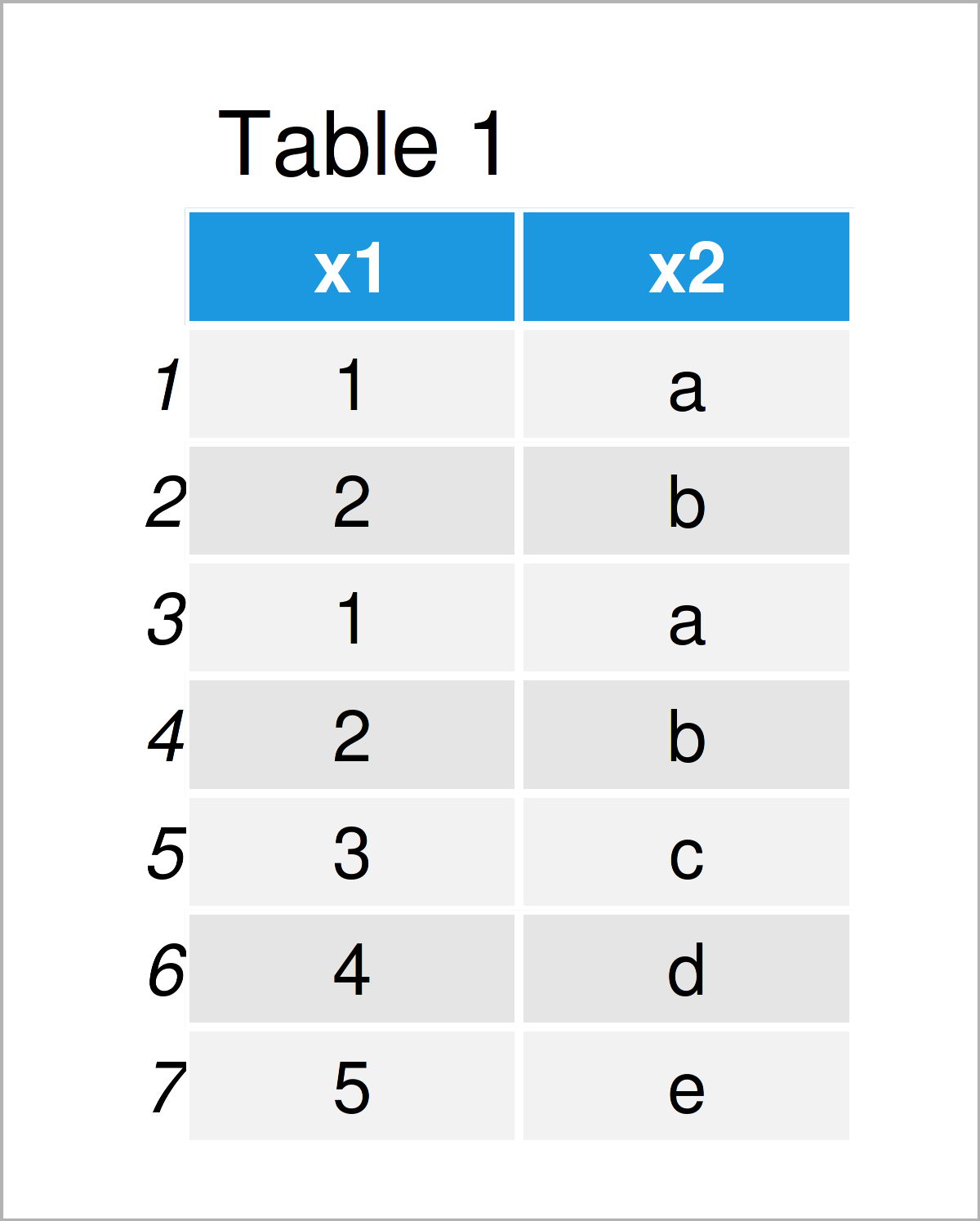# duplicated Function in R (2 Examples)

This article shows how to apply the duplicated function in the R programming language.

The page looks as follows:

If you want to know more about these content blocks, keep reading!

## Example 1: Apply duplicated() Function to Vector Object

In this example, I’ll demonstrate how to apply the duplicated function to a vector object.

First, we have to create an exemplifying vector in R:

```vec <- c("a", "b", "a", "b", "c")             # Create example vector
vec                                           # Print example vector
#  "a" "b" "a" "b" "c"```

The previous output of the RStudio console shows that our vector object contains five character elements. Two of those elements are duplicated.

We can systematically check that by applying the duplicated function to this vector:

```duplicated(vec)                               # Apply duplicated function
#  FALSE FALSE  TRUE  TRUE FALSE```

As you can see, a logical indicator has been returned that illustrates which of our vector elements are not unique. Note that the first occurrence of a non-unique element is set to FALSE, but the following non-unique elements are set to TRUE.

We may use this logical indicator to create a vector subset that contains only non-duplicated elements. For this, we have to specify a bang-sign (i.e. !) in front of the duplicated function:

```vec_unique <- vec[!duplicated(vec)]           # Subset unique values
vec_unique                                    # Print updated vector
#  "a" "b" "c"```

The previous R code has returned all unique values in our vector.

## Example 2: Apply duplicated() Function to Data Frame

In Example 2, I’ll illustrate how to apply the duplicated function to a data frame.

Let’s create some example data:

```data <- data.frame(x1 = c(1:2, 1:5),          # Create example data frame
x2 = letters[c(1:2, 1:5)])
data                                          # Print example data frame```Table 1 illustrates the structure of our example data frame. As you can see, the first two rows are the same as row numbers three and four.

We can use the duplicated command to return a logical vector that identifies those repeated rows:

```duplicated(data)                              # Apply duplicated function
#  FALSE FALSE  TRUE  TRUE FALSE FALSE FALSE```

We can now use this logical vector to subset all unique rows from our data:

```data_unique <- data[!duplicated(data), ]      # Subset unique rows
data_unique                                   # Print data with unique rows```As you can see in Table 2, all duplicated rows have been removed.

Based on the row names of Table 2 you can also see, that the later duplicates have been removed (i.e. rows 3 and 4). In case we want to remove the first duplicates (i.e. rows 1 and 2), we can use the fromLast argument as shown below:

```data_unique_last <- data[!duplicated(data,    # Using fromLast argument
fromLast = TRUE), ]
data_unique_last                              # Print data with unique rows```As you can see in Table 3, we have kept rows 3 and 4, and have deleted rows 1 and 2 instead. This can be useful in case you want to determine unique rows only based on some of the columns in a data frame.

## Video, Further Resources & Summary

I have recently published a video on my YouTube channel, which explains the R programming syntax of the present page. You can find the video below.

Please accept YouTube cookies to play this video. By accepting you will be accessing content from YouTube, a service provided by an external third party.If you accept this notice, your choice will be saved and the page will refresh.

Besides the video, you could have a look at the other tutorials on www.statisticsglobe.com. Some related tutorials are listed below:

In summary: At this point you should have learned how to use the duplicated command to determine, find, select, and extract duplicates in R. In case you have additional questions, let me know in the comments section below.

Subscribe to the Statistics Globe Newsletter